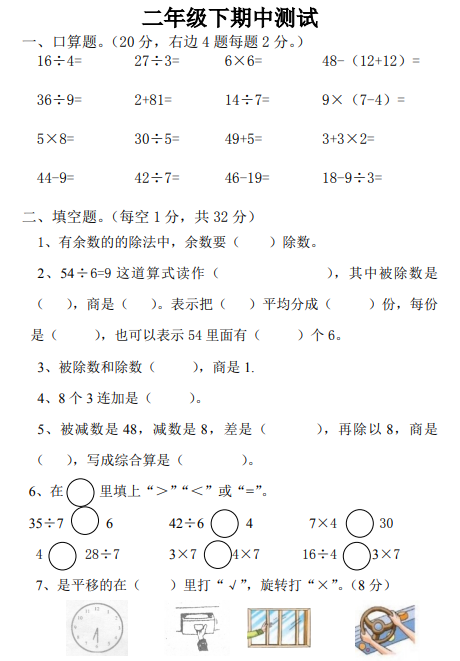16÷4= 27÷3= 6×6= 48-（12+12）=

36÷9= 2+81= 14÷7= 9×（7-4）=
5×8= 30÷5= 49+5= 3+3×2=
44-9= 42÷7= 46-19= 18-9÷3=

1、有余数的的除法中，余数要（ ）除数。

【PDF文档3页】新人教版小学二年级下学期数学期中试卷 (第五份)A4电子版资料_可直接打印_会员免费下载##### 评论信息